## Chapter 9Outlook and Conclusion

Even though several improvements of the SHE method are presented throughout this thesis, many possible further directions remain. In fact, the suggested improvements have lead to a number of direct follow-up research topics. Consequently, a discussion of possible further improvements on the SHE method is given in the next section. Finally, a conclusion is drawn.

### 9.1 Possible Further Improvements of the SHE Method

A lexicographically ordered list of possible improvements is briefly discussed in the following. The selection of topics is mostly based on an improvement of the method from the numerics point of view, because the author considers the method to be more mature from the physics point of view than from the numerics point of view.

#### 9.1.1 Bipolar SHE

The SHE method has so far been applied to either electrons or holes, but not both. The other carrier type has either been modelled by a continuity equation, or has been neglected. However, a distribution function for both carrier polarities is of interest for the investigation of high energy effects such as impact ionization feedback  , where highly energetic carriers interact with the crystal lattice and generate electron-hole pairs.

A challenging question is in the modeling of generation and recombination of carriers. While for a unipolar SHE the scattering operator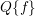acts on a single distribution function, a bipolar SHE requires the consideration of the distribution functions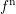andfor electrons and holes respectively, leading to a scattering operator of the form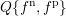. A Shockley-Read-Hall-like relaxation-time approach for the generation and regeneration of carriers can serve as a first approach to modelling the interactions of electrons and holes, but higher sophistication is expected to be required for modelling high-energy effects accurately.

#### 9.1.2 Energy Grid with Hanging Nodes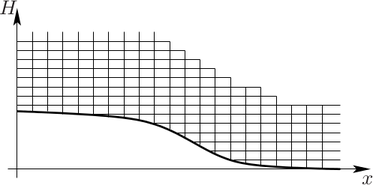(a) Truncated simulation domain.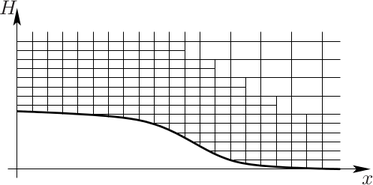(b) Hanging nodes.

Figure 9.1: Two possibilities for increasing the numerical efficiency of the SHE method by reducing the resolution in low-probability regions in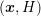-space.

Due to the numerical stabilization using the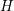-transform discussed in Sec. 2.3, a tensor-prolongation of the mesh in-space to the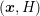-space is attractive for the discretization presented in Chap. 5. This prolongation for discrete total energies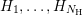is not fully satisfactory from the numerics point of view: The range of kinetic energies over the device varies by an amount of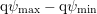over the device, where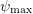and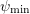denote the maximum and minimum of the electrostatic potential over the device. At low applied voltages, the difference in kinetic energy ranges is abouteV, which is certainly acceptable. However, for higher voltages above ten Volts, as it is common for high-power devices, the kinetic energy range consequently varies by more than ten electron volts. This can lead to a resolution of regions of the distribution function with extremely low probabilities of more than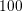orders of magnitude below the probability near the band edge. A resolution of these areas of extremely low probabilities is typically not required, hence computational resources should be focused on regions with higher importance.

Two possible remedies for the reduction of an unnecessary high resolution at high kinetic energies are depicted in Fig. 9.1. The first possibility is to simply truncate the simulation domain above a certain kinetic energy and impose homogeneous Neumann boundary conditions at the new boundaries. The truncation errors are certainly negligible if there is no significant high-energy tail of the distribution function extending into the the truncated region. Consequently, such a truncation is particularly interesting for larger devices, where the mean free path of carriers is much smaller than the device dimensions. However, for the case of small devices in the deca-nanometer regime, the high energy tail cannot be neglected and thus a truncation of the simulation domain is inappropriate. Nevertheless, the resolution at higher energies can be reduced by allowing so-called hanging nodes inside the mesh. In such an approach, the energy spacing is locally increased from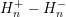to, say,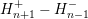. Hanging nodes can also be introduced by the use of nested grids, for which one may in addition decrease the spatial resolution, cf. Fig. 9.1(b). This would further lead to the use of multigrid methods   for the SHE method.

The difficulty of a mesh with hanging nodes is in the conservation of current. While the box integration scheme in Chap. 5 asserts current conservation for sufficiently regular Delaunay meshes by construction, it is not directly applicable for meshes with hanging nodes in-space. On the other hand, a violation of the conservation property in low-probability regions may be acceptable, because the error will be in the range of the machine precision due to a violation in low-probability regions only.

#### 9.1.3 Fast Self-Consistency with Poisson’s Equation

The nonlinear coupling of the BTE with the Poisson equation requires the use of iteration schemes as discussed in Sec. 2.4. Clearly, it is desirable to keep additional computational costs due to these nonlinear iterations as small as possible. For simplicity, the discussion is mostly based on uniform expansion orders, but equally applies to adaptive expansion orders presented in Chap. 6 as well.

For a SHE method with order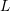, a considerable reduction of the total simulation time can be achieved by first computing a self-consistent solution of a first-order expansion, possibly using a coarser grid in-space, larger energy spacings in-space, or both. Since the BTE is coupled with the Poisson equation by the carrier densities only, which in turn depend on the zeroth-order SHE coefficient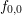only, a first-order SHE provides a very good initial guess for the SHE method of order. In particular, due to the quadratic convergence of the Newton method it is expected that a single Newton correction step for orderwhen using first-order SHE as initial guess is sufficient in most cases. While the savings are expected to be a factor of two to three for a third-order SHE, the savings for higher-order expansions will be roughly proportional to the number of nonlinear iterations required for a SHE of orderwithout improved initial guess for the potential.

The necessity for a nonlinear iteration scheme can directly be linked with adaptive expansion orders: As soon as the potential correction is sufficiently small, expansion orders can be increased. This procedure can be repeated until convergence and a prescribed accuracy is obtained. Even though the required adaptive expansion orders as well as error indicators and adaption strategies have been proposed in this thesis, a systematic study of the use within a nonlinear self-consistency iteration has not been carried out yet.

#### 9.1.4 More Flexible Discretization on Unstructured Grids

The discretization of the SHE equations on Delaunay triangulations as presented in Chap. 5 allows for a considerably better control of the local mesh size. However, the Delaunay criterion is hard or even impossible to fulfill for mesh hierarchies often used within multilevel and multigrid methods. As a consequence, it is desirable to employ discretization schemes suitable for arbitrary unstructured grids.

A possible replacement for the currently employed scheme based on Voronoi diagrams is the use of the barycenter method briefly outlined in Sec. 5.2. The discretization in Sec. 5.3 then needs to be adjusted for the surface integral over the generalized current density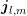. While each of the facets of a Voronoi box is perpendicular to the respective edge connecting two vertices, this is not the case for the barycenter method. This leads to additional effort, because the normal components of the fluxes with respect to the boxes are not directly available any longer.

An alternative to box integration schemes are mixed finite element schemes as well as discontinuous Galerkin methods, which have gained a lot of popularity recently  . Unlike the use of the barycenter method instead of Voronoi diagrams, a discretization for such a case still needs to be derived. Due to the complexity of the BTE, the derivation of a suitable formulation is expected to be considerably more involved than for well-behaved elliptic or parabolic equations.

#### 9.1.5 Preconditioner for the Compressed Matrix Scheme

In Chap. 4 it has been shown that the system matrix for the SHE equations can be written in the form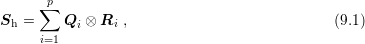where the number of terms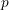depends on the band structure model employed. The attractiveness of this representation not only stems from the reduction of memory requirements from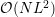to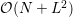, wheredenotes the number of nodes in thesimulation domain, but also from the ability to conveniently switch between different expansion orders. Denoting with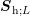the system matrix resulting from a SHE of the BTE of order, the system matrices for e.g.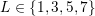would have to be set up separately each time. With (9.1), these system matrices are given by replacing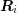with the respective expansion order.

The main disadvantage of (9.1) are the structural restrictions during the solution phase. Since preconditioners are required to obtain convergence of the iterative solvers, a suitable preconditioner still needs to be found for the representation (9.1). With the availability of adaptive expansion orders and the fact that the SHE method is basically limited by its memory requirements rather than its computational costs, the use of uniform expansion orders with a representation of the form (9.1) makes sense only if the preconditioner requires memory of order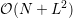or at most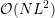with a small factor of proportionality in order to be comparable to the memory requirements of the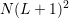unknowns. Otherwise, the use of adaptive expansion orders is likely to be the better choice.

The preconditioner scheme discussed in Chap. 7 requiresmemory with a considerably large constant of proportionality in front, which undermines the advantage of the compressed storage of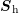. A possibility to reduce memory requirements tois to compute the preconditioner for first-order SHE as usual, and to define in similarity to algebraic multigrid methods suitable transfer operators for the restriction of the residual of the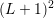expansion coefficients to the zeroth-order expansion coefficient, and the prolongation from the zeroth-order coefficient to the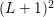expansion coefficients. However, it is not clear whether suitable transfer operators can be defined.

A different approach is based on an approximation on the algebraic level. Approximating first the the system matrix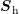by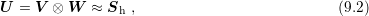the preconditioner can be computed from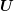by the use of the identity. Consequently, only a preconditioner for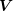and one for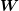needs to be computed, which then serves as a preconditioner forand thus for. Still, the question of whether such suitable approximationsto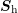exist, is still open.

### 9.2 Conclusion

Throughout this thesis a number of extensions to the SHE method are proposed in order to reduce the computational effort and consequently increase the attractiveness of the method for every-day TCAD purposes. The suggestions enable the simulation of semiconductor devices in the deca-nanometer regime at an unprecedented accuracy for a given time budget.

The increased importance of hot carrier degradation in modern scaled-down devices is addressed by proposing a scheme for the inclusion of carrier-carrier scatting for the SHE method. Unfortunately, the scheme leads to nonlocal coupling of the equations with energy, such that additional computational effort is required. In addition, the SHE equations become nonlinear, which is less a concern since the SHE equations are already nonlinearly coupled with the Poisson equation. Nevertheless, similar complications arise when considering carrier-carrier scattering with the Monte Carlo method.

A thorough investigation of the coupling structure of the SHE equations leads to a matrix compression scheme, which allows for storing the system matrix of the linear system of equations after discretization in a very efficient manner. Even though the method greatly reduces memory requirements, its use is so far hampered by the need for a suitable preconditioner for the iterative solvers. Nevertheless, the method can already be employed in the cases where convergence is obtained without preconditioner, and for the evaluation of residuals for adaptive expansion order strategies.

The formulation and implementation of a higher-order discretization scheme for unstructured grids enables the use of the SHE method as a direct replacement for established macroscopic transport models such as the drift-diffusion model or the hydrodynamic model without changing the underlying mesh. Moreover, the number of unknowns compared to structured grids is reduced considerably, because refinement in unstructured grids leads to a much more localized reduction of the mesh size than for structured grids, especially in three spatial dimensions.

Variable-order expansions achieve the accuracy of uniform expansions at considerably lower computational cost. The extraction of macroscopic quantities such as the carrier velocity or the average carrier energy are shown to require only locally increased expansion orders in the high-probability regions of the distribution function. Three different types of adaptive strategies for the automatic choice of the expansion order are proposed.

To address the shift towards parallel computing architectures, a parallel preconditioner scheme for the SHE method is derived based on physical principles. The resulting block-preconditioner scheme is shown to be able to use modern multi- and many-core computing architectures such as CPUs and GPUs efficiently. Performance gains of up to one order of magnitude compared to single-threaded executions are obtained.

On the overall, the novel methods presented in this thesis allow for a reduction of execution times for device simulations employing the SHE method by up to two orders of magnitude compared to existing approaches. In addition, memory requirements are reduced by about one order of magnitude, thus allowing for higher accuracy for a given amount of memory. The proposed algorithms are implemented in the freely available open source device simulator ViennaSHE.